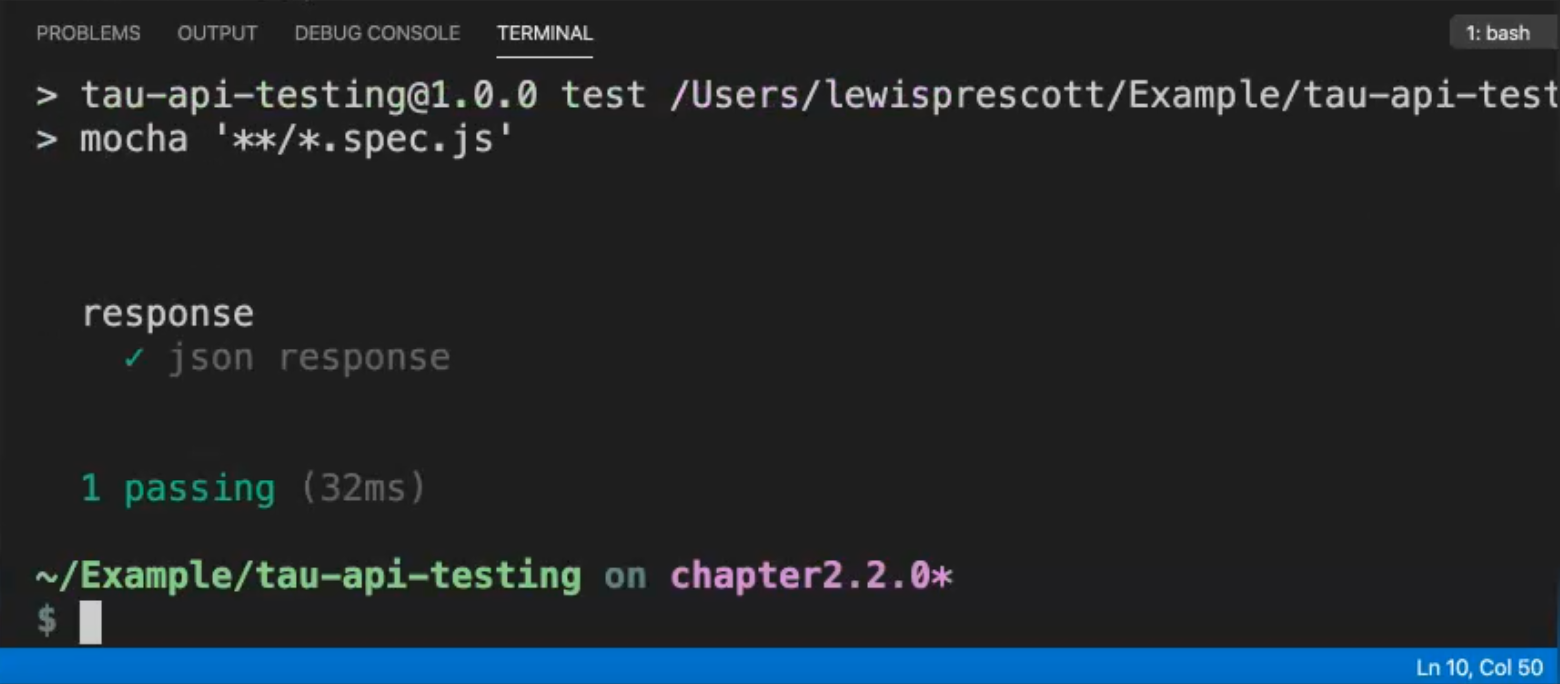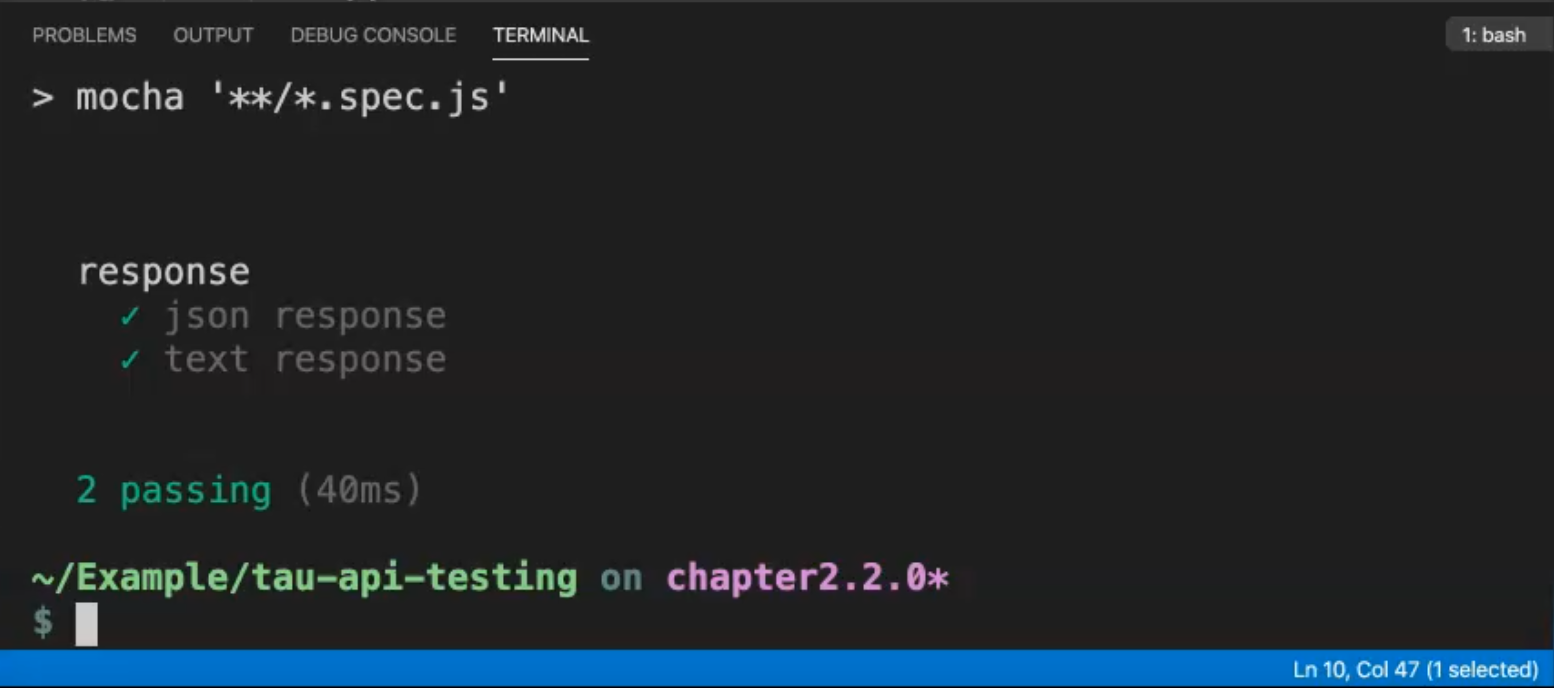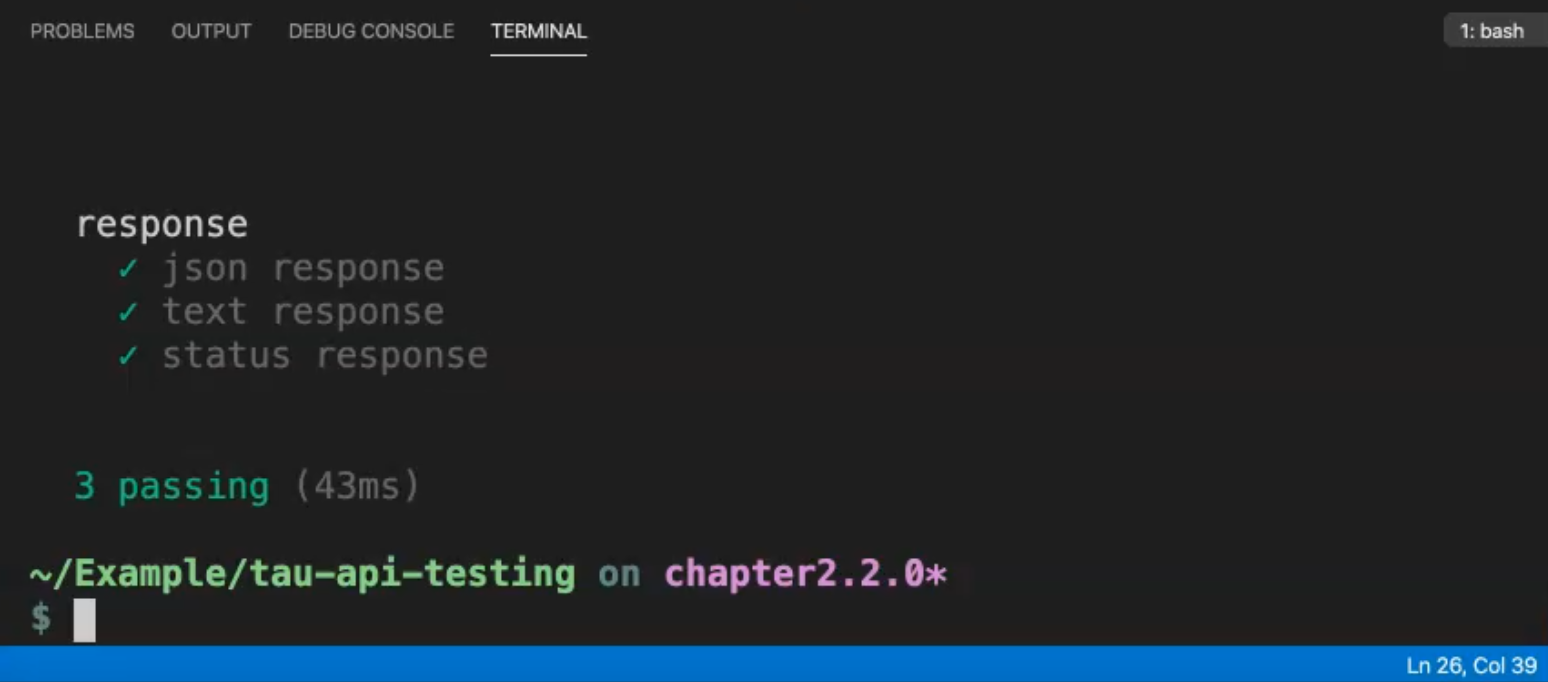Transcripted Summary

Now in this chapter, we're going to be looking at the response in more detail in `response.spec.js`.

Using SuperTest, there's a couple of different formats that we can use for the response, such as the text and the JSON object.

Also, we can assert the status code directly from the response.

So I'll show you all these things in this chapter.

### # JSON Response

First, we're going to use the default JSON response.

In our first test, we're going to check the JSON response body.

As seen in previous chapters, we can directly use the `body`.

This is because the `body` is directly passed using SuperTest, so we don't need to worry about converting the object into the format that we want.

So when we make the `request` to the `app` to get the course, we can `expect` when the response is returned, that the `body` is available to be chained directly to the response, and in doing this, we can check that the `id` in this example is equal to `1`.

``````const app = require('../src/app');
const request = require('supertest');
const expect = require('chai').expect;

describe('response', () => {
it('json response', () => {
request(app)
.get('/course')
.end((err, res) => {
expect(res.body.id).to.be.equal('1');
});
});
});
``````

As you can see here, the test is passing.### # Text Response

So in the next test we're going to be looking at text.

Maybe when you're asserting your response, you don't need to worry about the structure of the object, such as in the JSON response.

You just want to assert that the response has the correct value or the correct contents.

Let's look at a text response in SuperTest.

Again, this is directly converted for you into a string object, so you don't need to worry about doing any conversions.

If we look at getting the course again, on the response itself, we can get `res.text` and this will give us a string.

Then we can assert that it contains the value that we want.

``````const app = require('../src/app');
const request = require('supertest');
const expect = require('chai').expect;

describe('response', () => {
it('json response', () => {
request(app)
.get('/course')
.end((err, res) => {
expect(res.body.id).to.be.equal('1');
});
});

it('text response', () => {
request(app)
.get('/course')
.end((err, res) => {
expect(res.text).to.contain('1');
});
});
});
``````

If we run this again, we can see the text response contains the value `1`.So we didn't need to understand that it was within the `body` and within the `id` - we can just check on the whole text object.

### # Status Response

The third thing we're going to do in this module is, we're going to assert the status directly on the response.

Let's add a test for the status.

So when we `request` the `app` and we `get` the `/course`, we just want to verify that the response returns us an `ok` response.

We can do that directly like this - `expect(res.ok).to.be.equal(true)` and the `ok` response returns us a boolean value.

When asserting a boolean value, we can write it like this, or instead we can just use the short version `expect(res.ok).to.be.true`.

This reads much better than passing in a 200 response, because someone who would like to read the test can understand that it's an `ok` response, instead of having to understand the HTTP code itself.

``````const app = require('../src/app');
const request = require('supertest');
const expect = require('chai').expect;

describe('response', () => {
it('json response', () => {
request(app)
.get('/course')
.end((err, res) => {
expect(res.body.id).to.be.equal('1');
});
});

it('text response', () => {
request(app)
.get('/course')
.end((err, res) => {
expect(res.text).to.contain('1');
});
});

it('status response', () => {
request(app)
.get('/course')
.end((err, res) => {
expect(res.ok).to.be.true;
});
});
});
``````

So now if we run this test, we can see that the "status response" has passed.That's it for this module and in the next module we'll be looking at mocking out some of the responses using Nock.

So what have we learned in this module?

We've learned that the object is directly passed using SuperTest, and we can interact with the text object, not only the JSON one.

Also, the response code is passed to us in a readable format, so we can assert against the boolean of the status code.